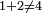# Perfect number

In mathematics, a perfect number is a positive whole number whose proper divisors sum to the number itself. For instance, 6 is a perfect number, as the proper divisors of 6 are 1, 2, and 3, and 1+2+3=6. In contrast, 4 is not a perfect number, as the proper divisors of 4 are 1 and 2, and$1+2 \neq 4$. Although the name perfect suggests that these integers have some importance, they have remained little more than a curiosity since the name was coined thousands of years ago. Still, the study and search for perfect numbers has occupied and fascinated many influential mathematicians, notably Euclid, Fermat, and Euler. Also, the search for perfect numbers is still ongoing indirectly through the Great Internet Mersenne Prime Search (GIMPS), and the search for and enumeration of the properties of odd perfect numbers remains a topic of current research.

## History

The origin of the term "perfect number" appears to be unknown. Ancient Greeks probably ascribed mystical properties to these numbers. Other cultures have held similar numerological beliefs, and other types of numbers have been called perfect. The above definition of perfect numbers eventually became dominant, and is the sole definition accepted by most current mathematicians.

Euclid's Elements comprises 13 books, and books 7, 8, and 9 cover arithmetic. The earliest historical definition of a perfect number is probably the one near the beginning of book 7, and no further mention is made of them until the final proposition of book 9. In this proposition, Euclid showed that if n is a whole number for which 2n − 1 is prime, then the number

2n − 1(2n − 1)

is perfect. For instance, setting n=2, the number 22 − 1 = 3 is prime. Euclid's result thus applies to this particular value of n, and using his formula we recover the perfect number

22 − 1(22 − 1) = 6.

Prime numbers of the form 2n − 1 also occur in other arithmetical contexts, and are now referred to as Mersenne primes. The first few exponents for which the number 2n − 1 is prime are n=2, 3, 5, and 7, and putting these into Euclid's formula gives the first four perfect numbers, 6, 28, 496, and 8128. Note that Euclid did not state that every perfect number can be produced by his formula, so we cannot know that these are the four smallest perfect numbers without directly checking that there are no other perfect numbers smaller than 8128.

Many historians have suggested that Euclid's positioning of his result at the end of the arithmetical books of Elements, even past his proof of the infinitude of prime numbers, indicates that Euclid believed this the culmination of the arithmetical work in Elements. As Elements remains the most influential mathematical text in history, it is certain that myriad aspiring mathematicians since have pondered how to produce more perfect numbers.

The next significant result in this direction occurred only in the eighteenth century, when Euler proved that the only perfect numbers which are also even are those provided by Euclid. (A proof of this can be found on the "advanced" subpage for this page.) Together, Euclid and Euler reduced the problem of finding even perfect numbers to the problem of finding Mersenne primes. This is an ongoing search, and most notably, the Great Internet Mersenne Prime Search (GIMPS) is a distributed computing effort to harness the computing power of computers around the world in discovering new Mersenne prime numbers. With each new such prime that is discovered, a new perfect number is discovered as well. It is not presently known if there are infinitely many such primes, or equivalently, infinitely many perfect numbers.

## Odd perfect numbers

As mentioned above, the even perfect numbers have been completely classified. In contrast, despite intense efforts, no odd perfect number has ever been discovered, and no proof has been found to show that no odd perfect numbers exist.

## Related types of numbers

Since Euclid's time, many special types of numbers with definitions similar to the definition of perfect numbers have been investigated. See the Related pages subpage for links to these other types of numbers.Some content on this page may previously have appeared on Citizendium.